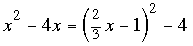SEARCH HOMEMath Central Quandaries & QueriesQuestion from Yumiko, a student: Solve the following pair of simultaneous equations. x^2 -4x = y^2-4 3y=2x - 3Yumiko,

Solve the second equation for y and substitute into the first equation to getExpand the right side, multiply both sides by 9 to clear the fractions, simplify and factor.

PennyMath Central is supported by the University of Regina and The Pacific Institute for the Mathematical Sciences.